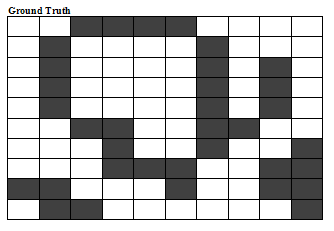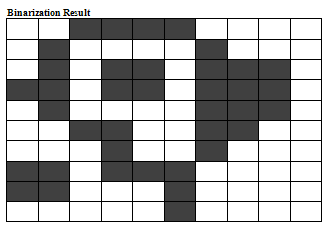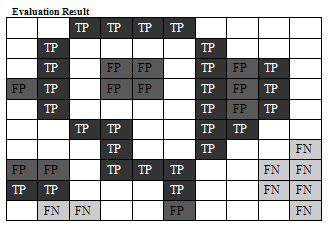DIBCO 2009 - Document Image Binarization Contest
(in the framework of ICDAR2009)

Evaluation:

 The performance evaluation will be based on a well established technique that counts True Positive (TP), False Positive (FP) and False Negative (FN) pixels in order to calculate Recall and Precision metrics.  A pixel is classified as True Positive (TP) if it is ON in both the GT and the Binarization Result images.A pixel is classified as False Positive (FP) if it is ON only in the Binarization Result image.A pixel is classified as False Negative (FN) if it is ON only in the GT image. If CTP the number of TP pixels, CFP the number of FP pixels and CFN the number of FN pixels, Recall (RC) and Precision (PR) metrics are given as follows:RC = CTP/(CFN+CTP)PR = CTP/(CFP+CTP)The overall metric that will be used for ranking is the F-Measure (FM) which is calculated as follows:FM =  (2xRCxPR / (RC+PR)) x 100 % Example:CTP = 27, CFP = 11, CFN = 8, RC = .77, PR = .71, FM = 73.9%

Site maintained by B. Gatos, email: bgat<at>iit<dot>demokritos<dot>gr
Last update: 19/12/2008